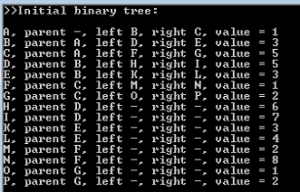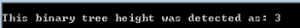# Minimax Alpha Beta Pruning Example: Part 1

Create a binary tree of 15 nodes which use the following names:

```
[A]
/        \
[B]            [C]
/   \         /    \
[D]    [E]     [F]    [G]
/ \     / \    /  \    /  \
[H] [I] [J] [K] [L] [M] [N] [O]
```

with the nodes holding the following values:

```

/       \
            
/    \          /   \
               
/ \     / \     / \     / \
       
```
• The binary tree must be created automatically from an array containing the values: {1,3,5,5,3,1,2,6,7,3,4,2,8,1,2}.
• Display the tree in console window showing the following information: node name, parent node name, children node names, and the value stored in each node. Hyphenate the non-existing parent/children nodes.
• Calculate and display the height of the binary tree.

SOLUTION:
In this sample we will deal with a tree structure consisting from nodes. Each node can have a parent node, and (since its Binary tree) two children nodes: left and right. Each node also has a name, and a value it carries. Node class will look as following:

``` 	 	public class Node
{
public Node left { get; set; }
public Node right { get; set; }
public Node parent { get; set; }
public string name { get; set; }
public int value { get; set; }
public Node(Node p, string n, int v)
{
parent = p;
name = n;
value = v;
}
}
```

The pre-requisites for creation of our binary tree include a pair of arrays (one housing values and the other names of nodes):

```//Array of binary tree node values:
int[] arr = new int[] { 1,  3, 5,  5, 3, 1, 2,  6, 7, 3, 4, 2, 8, 1, 2 };
//Array of letter markers for discerning the nodes easier:
string[] arr2 = new string[] { "A", "B","C", "D","E","F","G", "H","I","K","L","M","N","O","P" };
```

Some other pre-requisites:

``` 	 	//Array which will house the number of nodes required:
Node[] tree = new Node[arr.Length];
//Nonexisting parent of the very first node in the tree:
Node parent = null; //We will use this variable to store the parents of subsequent nodes as well
```

We will go through the “tree” array, creating the nodes. To create the node, we need to provide a parent node, name, and numerical value respectively:

``` 	 	tree[i] = new Node(parent, arr2[i], arr[i]);
```

But we should take into account that the “null” parent is only fitting for the very first “parentless” node “A”. For all subsequent nodes, we need a mechanism that will assign proper parents to the next branches of 2, 4, 8 nodes and so on:

```if (i > 0) parent = tree[(i + (i % 2 == 0 ? 0 : 1) - 2) / 2];
```

Which makes for the following array-filling code:

```for (int i = 0; i < arr.Length; i++)
{
if (i > 0) parent = tree[(i + (i % 2 == 0 ? 0 : 1) - 2) / 2];
tree[i] = new Node(parent, arr2[i], arr[i]);
}
```

We are using arr.Length and not tree.Length since all of the arrays in this application have same length anyway. At this moment, we should have 15 nodes with “parent” attribute being filled. However, we have no children nodes yet. To fix this, we need to iterate through this array once again:

```//setting children nodes now that the every required node was created
for (int i = 0; i < arr.Length; i++)
{
//the last half of nodes lacks further children nodes, so they are set to null
tree[i].left = (i < arr.Length / 2) ? tree[i + i + 1] : null;
tree[i].right = (i < arr.Length / 2) ? tree[i + i + 2] : null;
}
```

Now we need to display the binary tree (which we will do on node-per-line basis), and for this we will create the appropriate function:

```static void ShowNodeData(Node n)
{
Console.Write("{0}, ", n.name);
if (n.parent != null) Console.Write("parent {0}, ", n.parent.name);
else Console.Write("parent -, ");
if (n.left != null) Console.Write("left {0}, ", n.left.name);
else Console.Write("left -, ");
if (n.right != null) Console.Write("right {0}, ", n.right.name);
else Console.Write("right -, ");
Console.WriteLine("value = {0}", n.value);
}
```

Calling this function from main part of application for every node in our array allows to display data about the whole binary tree:

```//displaying the binary tree
Console.WriteLine(">>Initial binary tree:");
Console.WriteLine();
foreach (Node item in tree)
{
ShowNodeData(item);
}
```

Result:The next function deals with finding the height of the tree, and uses recursion:

```static int findHeight(Node aNode)
{
if (aNode == null)
{
return -1;
}

int lefth = findHeight(aNode.left);
int righth = findHeight(aNode.right);

if (lefth > righth)
{
return lefth + 1;
}
else
{
return righth + 1;
}
}
```

Calling this function:

```WriteLine("This binary tree height was detected as: {0}", findHeight(tree));
```

results in:Therefore, we have successfully managed to create and display a binary tree, as well as calculate it’s height.

This is a first part of a minimax alpha beta pruning example created by our experts to help you with your assignment. We remind you that you are not allowed to use this project or any parts of it as your own without proper acknowledgements. If you need help with your programming project, place an order and let our professionals take care of your assignment. We guarantee you top-notch quality and 100% personal information security. Read our blog to find other parts of minimax alpha beta pruning example and other assignments on different topics and subjects.

Here you can read the next part of this sample – https://assignmentshark.com/blog/minimax-alpha-beta-pruning-example-part-2/.